Function Repository Resource:

# MultiPerspectiveEmbedding

Compute a 3D embedding for multiple graphs so that all pairwise distances are preserved simultaneously along various 2D projections

Contributed by: Nikolay Murzin
 ResourceFunction["MultiPerspectiveEmbedding"][gs] gives multi-perspective 3D embedding of graphs gs. ResourceFunction["MultiPerspectiveEmbedding"][ds] gives multi-perspective 3D embedding for explicit distance matrices.

## Details and Options

The Graph inputs gs should have the same set of vertices.
Distance matrices ds should be square and of the same dimensions.
ResourceFunction["MultiPerspectiveEmbedding"] accepts the following options:
 "InitialEmbedding" Automatic array of initial embedding vectors "InitialProjection" Automatic array of initial normal vectors "FixProjection" False whether to fix or learn projection vectors "UseGraphEmbedding" True if the input is graphs, whether to use its embeddings for computing distances "NormalizeDistances" True whether to normalize distances "NetTrainOptions" {} custom options for NetTrain TrainingProgressReporting Automatic use "Verbose" for custom reporting
This is an elementary layer for training embeddings X, with one projection normal vector Q and a distance matrix D.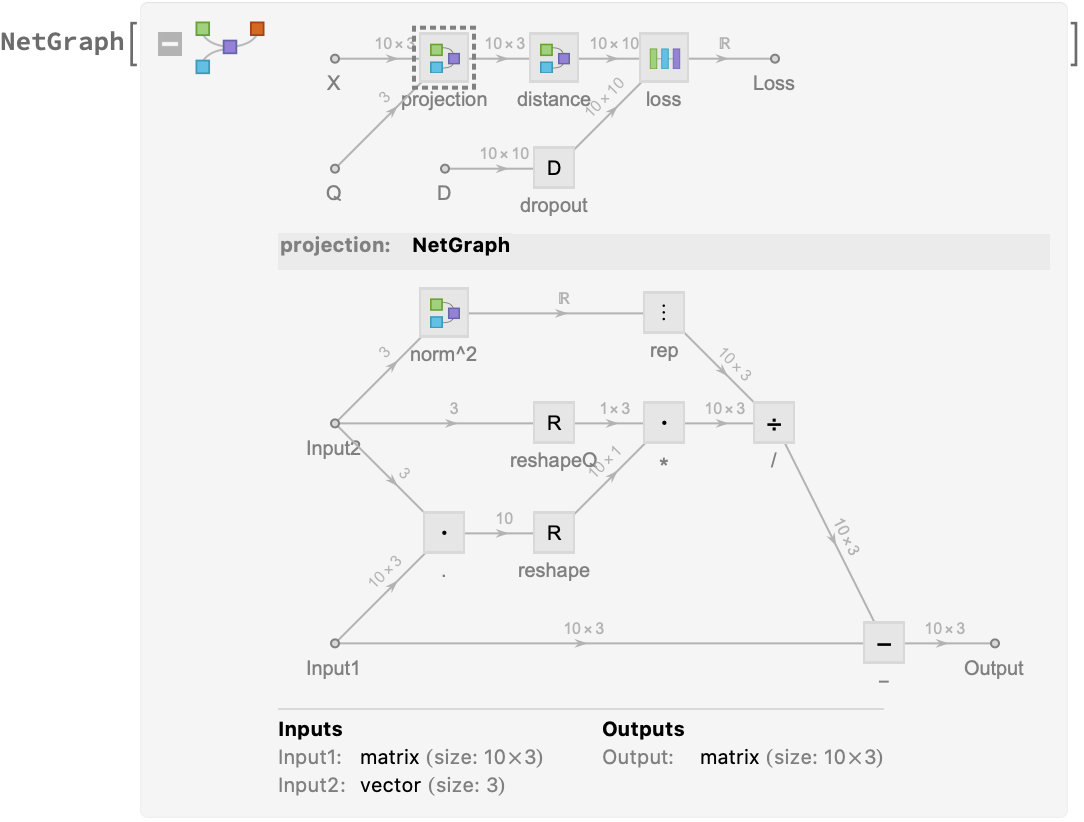## Examples

### Basic Examples (3)

Compute a 3D embedding for a grid graph and a path graph:

 In:=Out=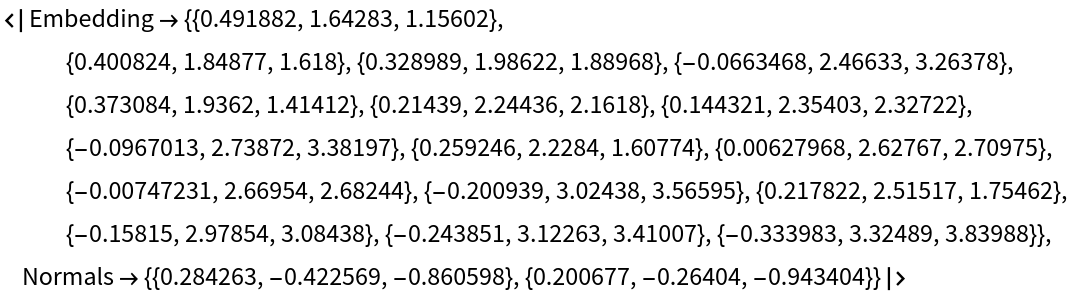Define larger grid and path graphs and show them:

 In:=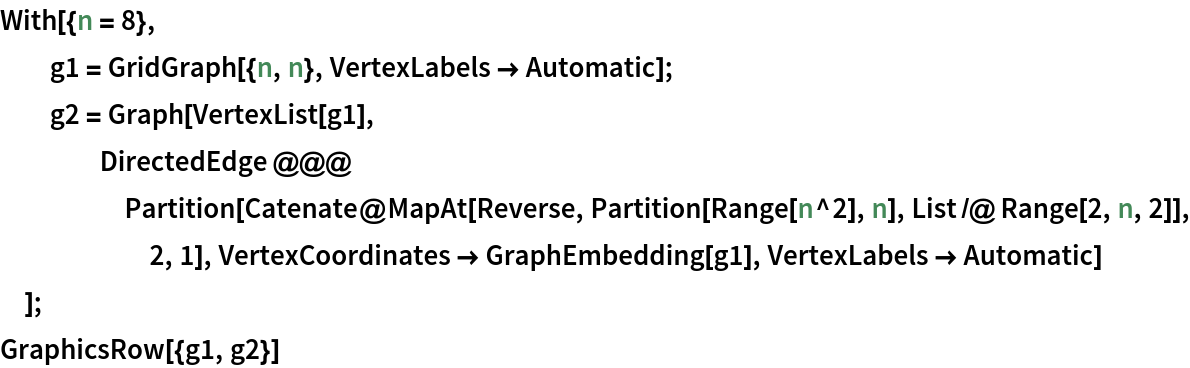Out=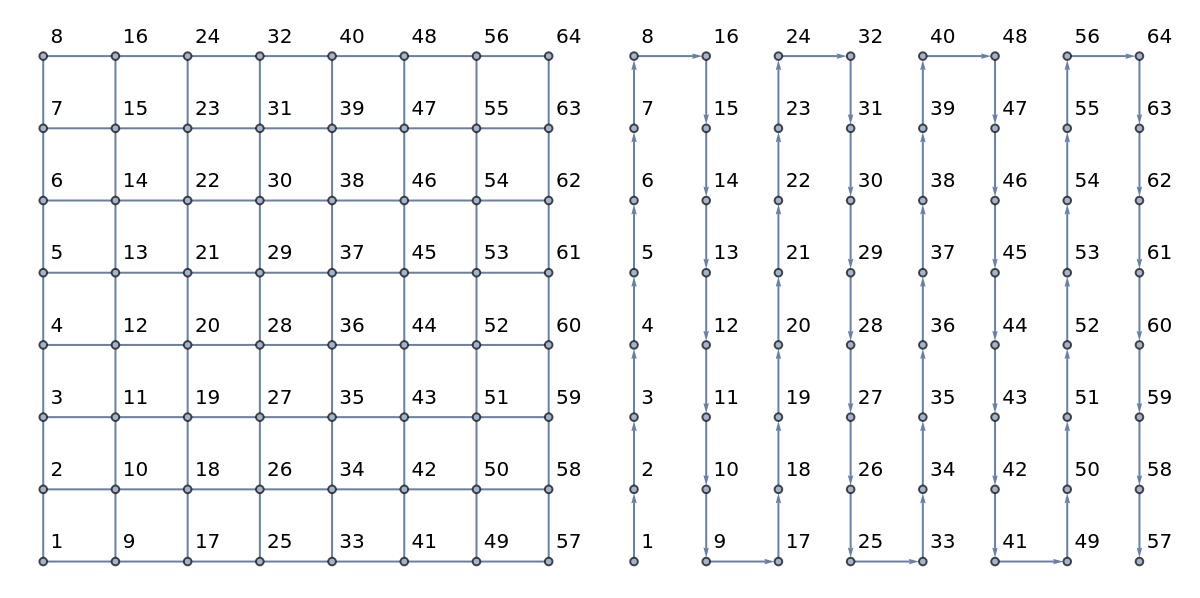Compute an embedding:

 In:=Out=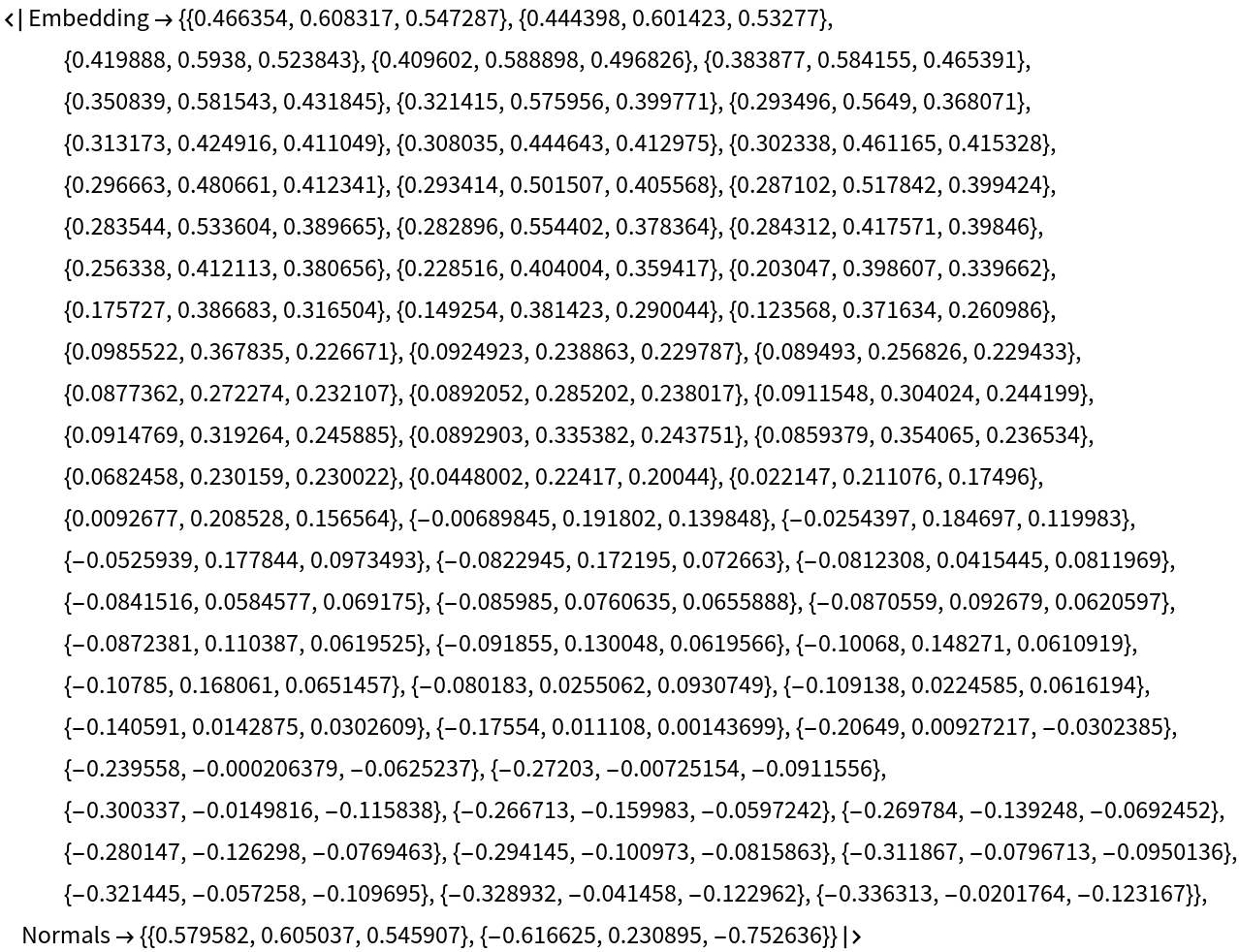View the embedding with the resource function MultiPerspectiveEmbeddingViewer:

 In:=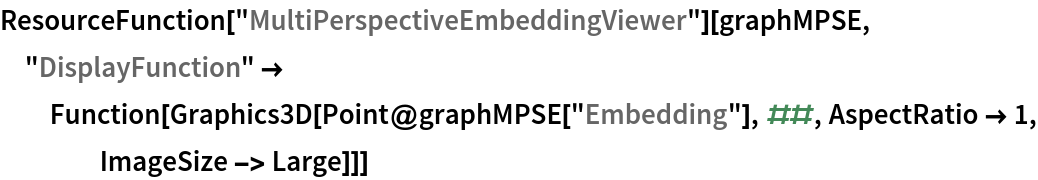Out=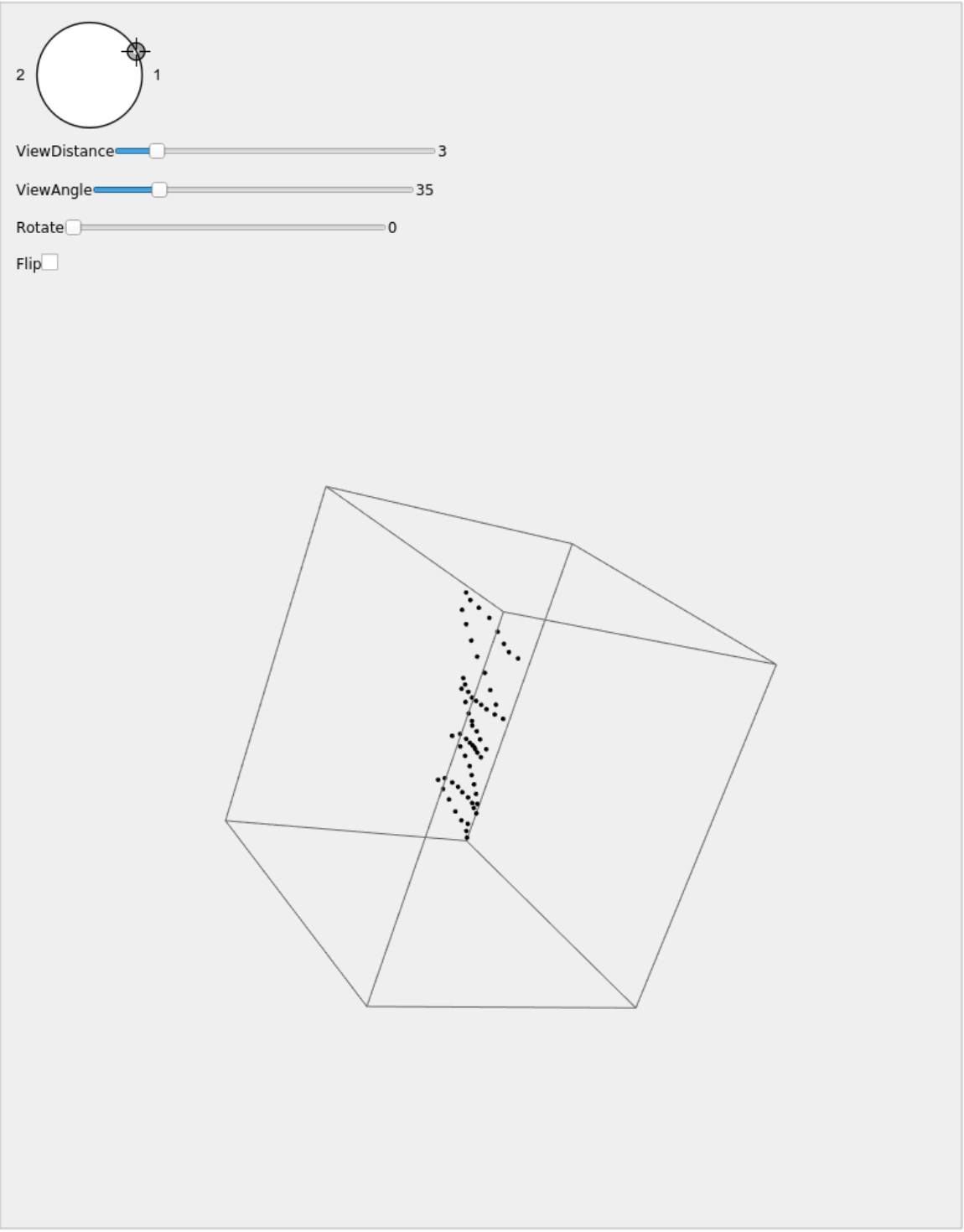Here is the progress for training an embedding for points that look like digits 1, 2 and 3 in 2D from three distance matrices and how they form into their position:

 In:=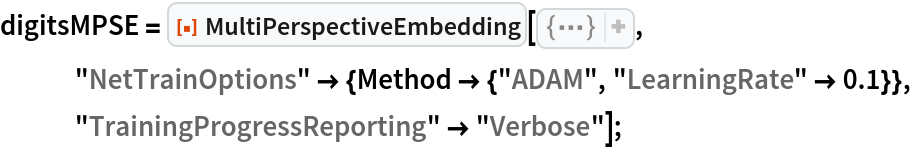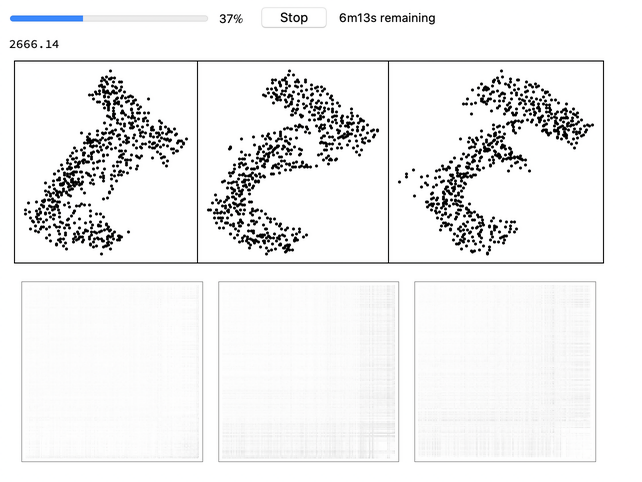View the embedding:

 In:=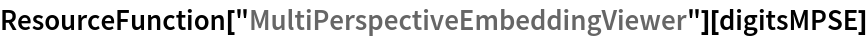Out=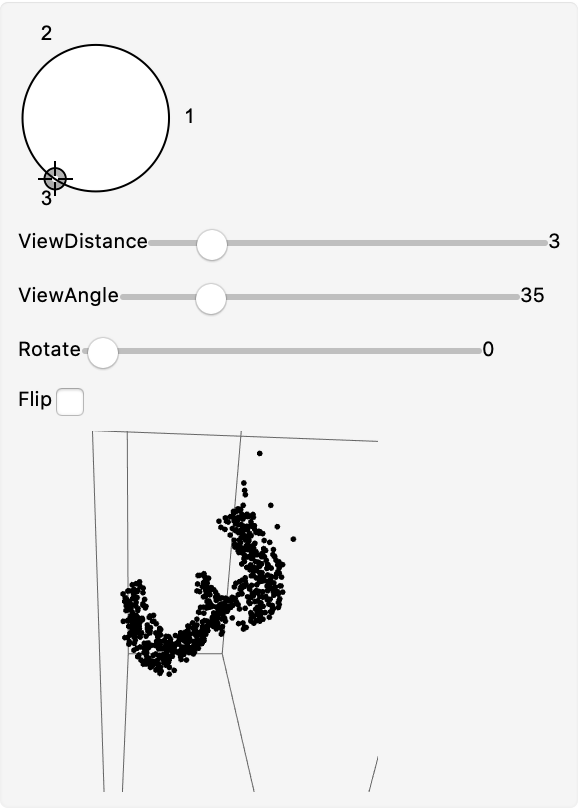### Options (7)

#### NetTrainOptions (1)

Specify custom options for NetTrain, for example, MaxTrainingRounds:

 In:=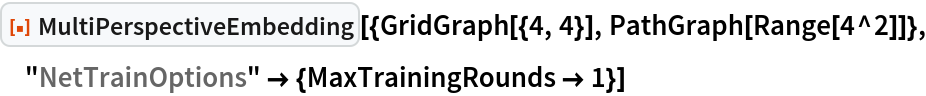Out=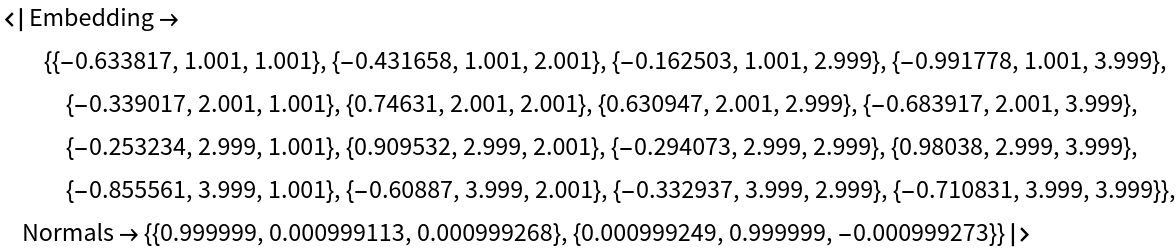#### InitialEmbedding (1)

Specify an initial embedding:

 In:=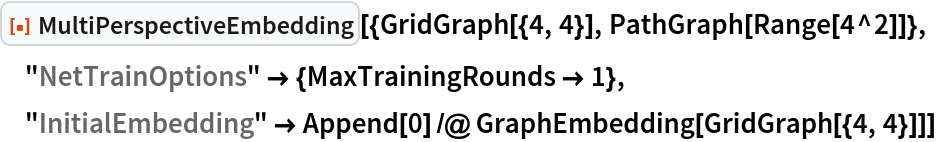Out=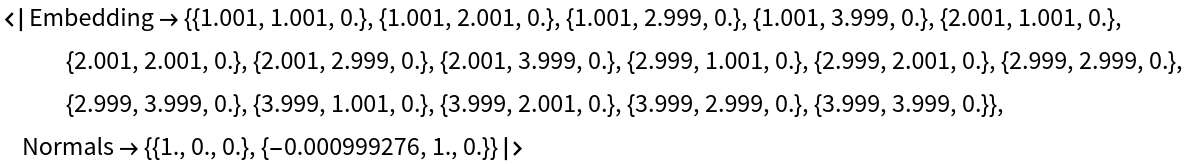#### InitialProjection (1)

Specify initial normal projection vectors using the "InitialProjection" option. The "Normals" key of the resulting association would contain new projection normal vectors after training:

 In:=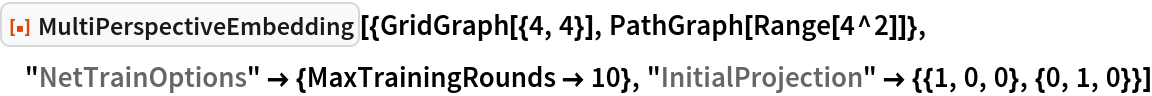Out=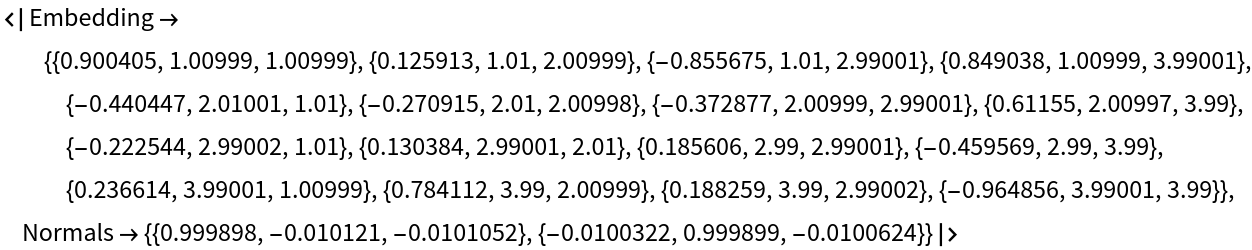#### FixProjection (1)

Disable learning for normal vectors. The "Normals" value stays the same:

 In:=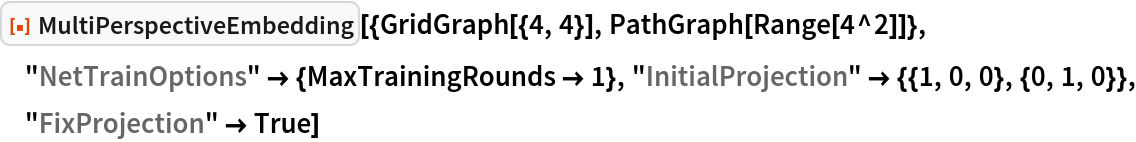Out=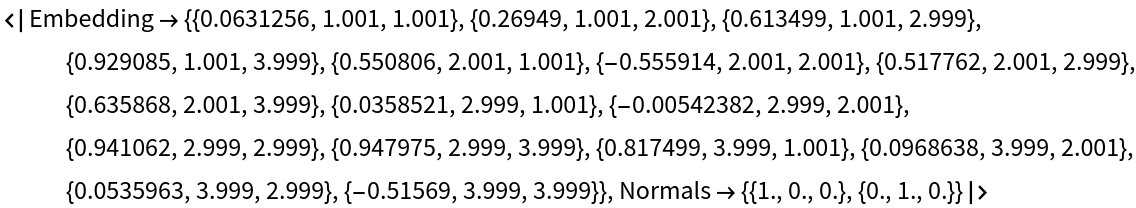#### UseGraphEmbedding (2)

By default, distances are taken to be the distances between its graph embeddings:

 In:=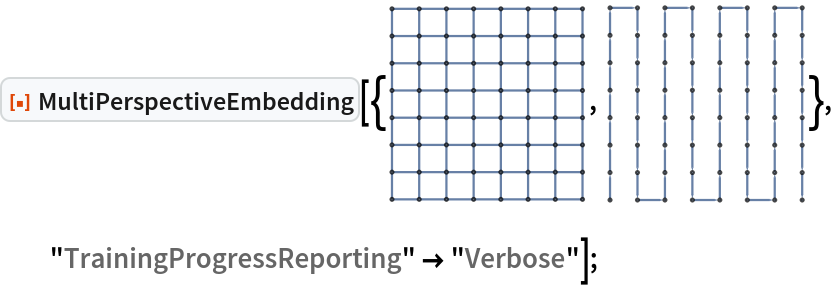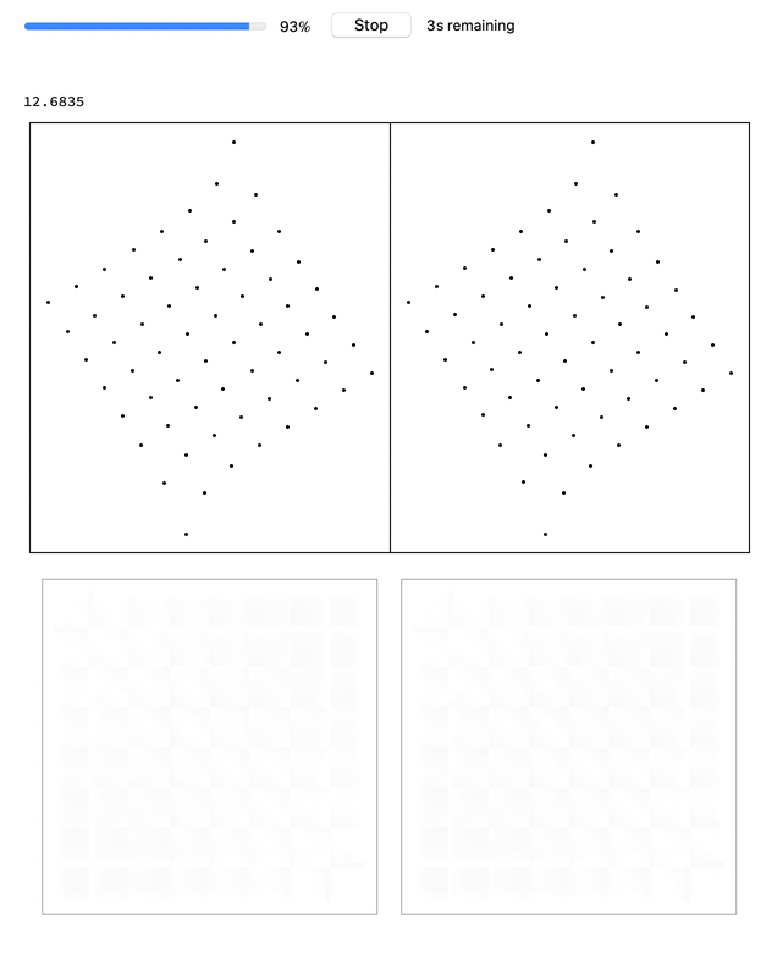UseGraphEmbedding False would use GraphDistanceMatrix instead:

 In:=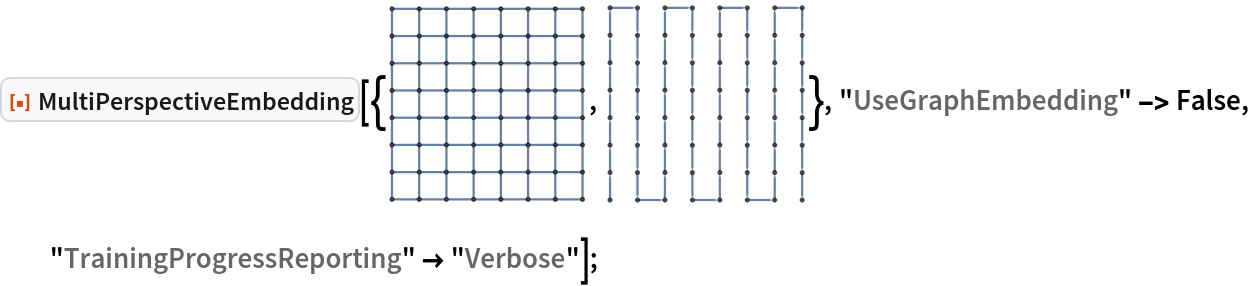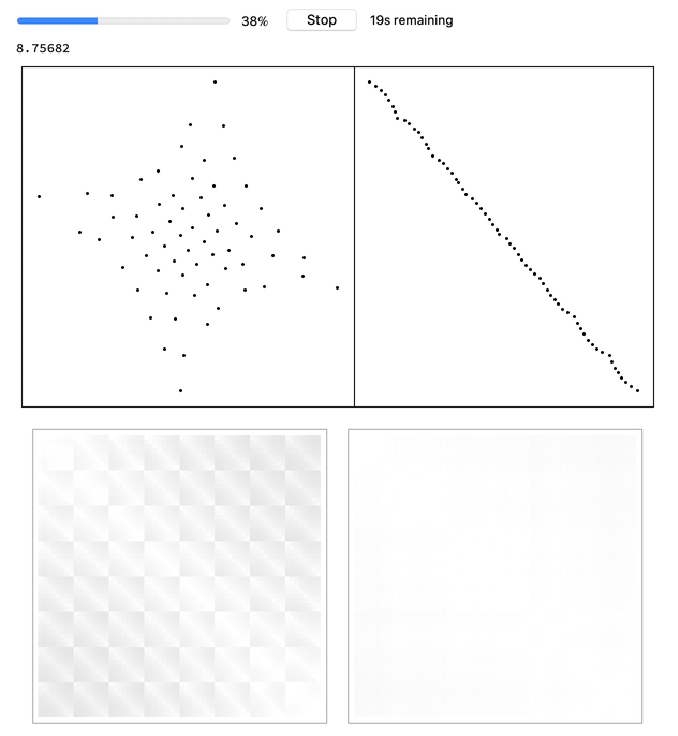#### NormalizeDistances (1)

Without normalizing distances, training may diverge:

 In:=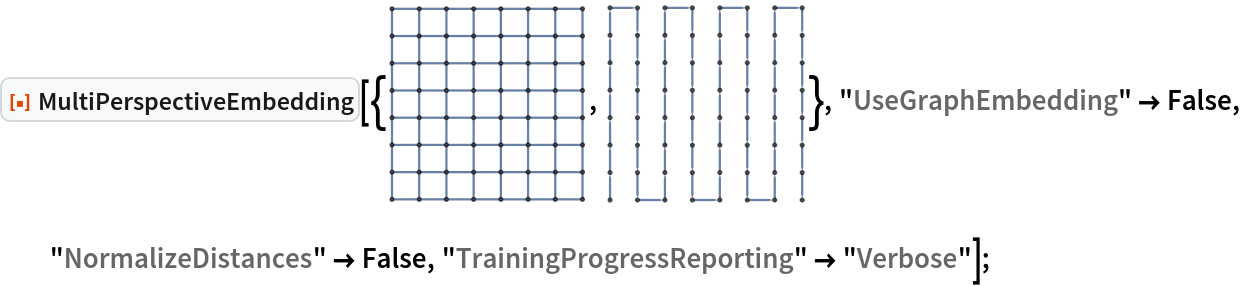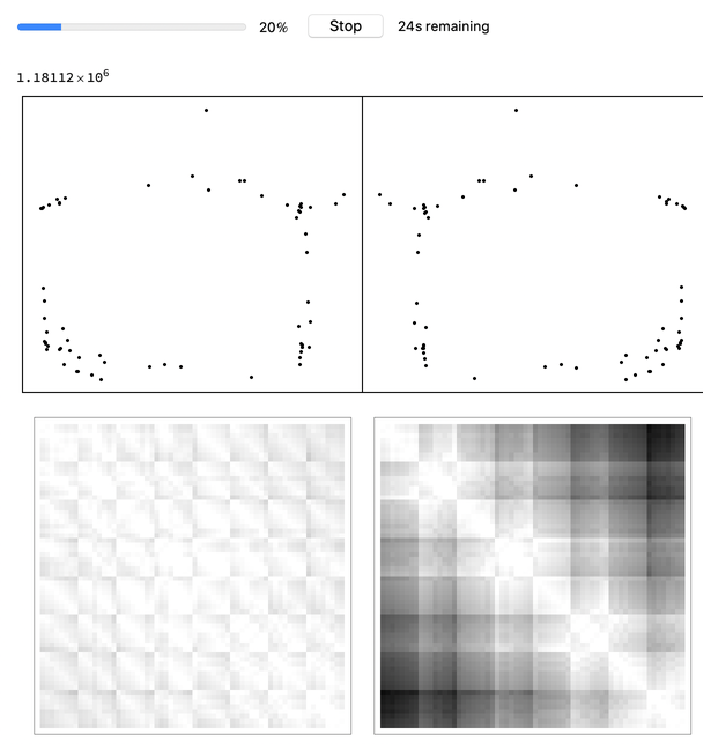N. Murzin

## Version History

• 2.0.0 – 21 January 2021
• 1.0.0 – 17 July 2020